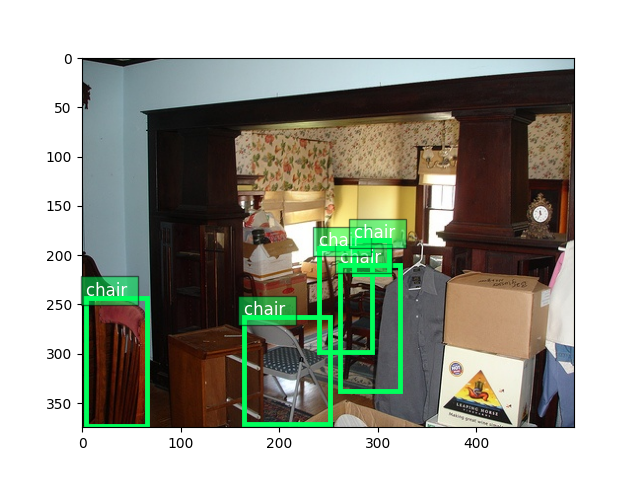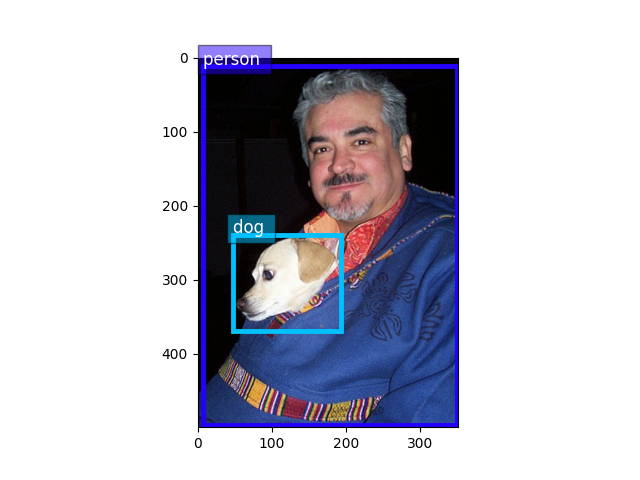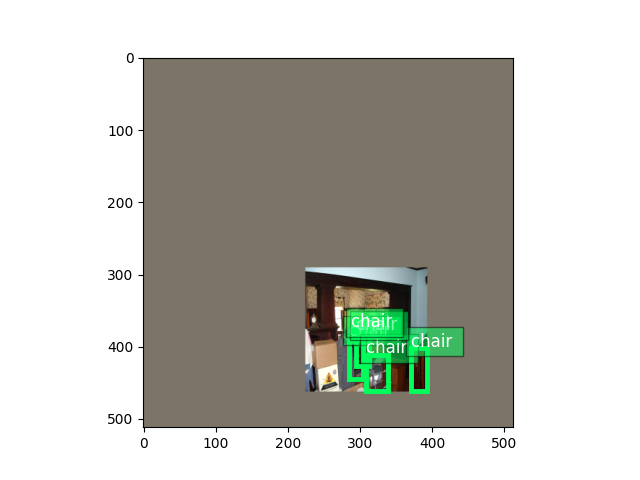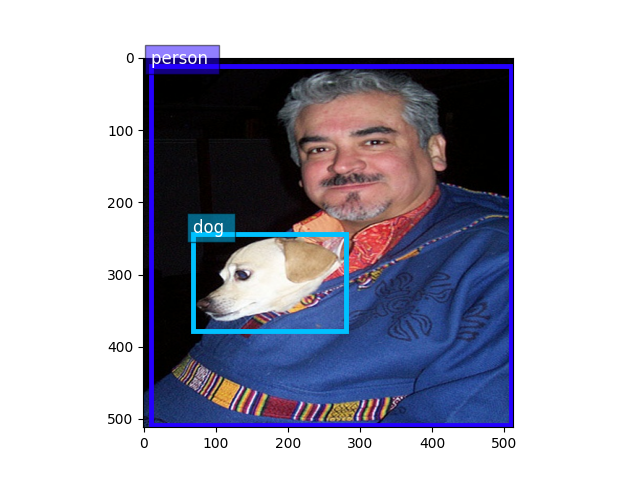# 04. Train SSD on Pascal VOC dataset¶

This tutorial goes through the basic building blocks of object detection provided by GluonCV. Specifically, we show how to build a state-of-the-art Single Shot Multibox Detection [Liu16] model by stacking GluonCV components. This is also a good starting point for your own object detection project.

Hint

`Download train_ssd.py`

Example usage:

Train a default vgg16_atrous 300x300 model with Pascal VOC on GPU 0:

```python train_ssd.py
```

Train a resnet50_v1 512x512 model on GPU 0,1,2,3:

```python train_ssd.py --gpus 0,1,2,3 --network resnet50_v1 --data-shape 512
```

Check the supported arguments:

```python train_ssd.py --help
```

## Dataset¶

Please first go through this Prepare PASCAL VOC datasets tutorial to setup Pascal VOC dataset on your disk. Then, we are ready to load training and validation images.

```from gluoncv.data import VOCDetection
# typically we use 2007+2012 trainval splits for training data
train_dataset = VOCDetection(splits=[(2007, 'trainval'), (2012, 'trainval')])
# and use 2007 test as validation data
val_dataset = VOCDetection(splits=[(2007, 'test')])

print('Training images:', len(train_dataset))
print('Validation images:', len(val_dataset))
```

Out:

```Training images: 16551
Validation images: 4952
```

## Data transform¶

We can read an image-label pair from the training dataset:

```train_image, train_label = train_dataset
bboxes = train_label[:, :4]
cids = train_label[:, 4:5]
print('image:', train_image.shape)
print('bboxes:', bboxes.shape, 'class ids:', cids.shape)
```

Out:

```image: (375, 500, 3)
bboxes: (5, 4) class ids: (5, 1)
```

Plot the image, together with the bounding box labels:

```from matplotlib import pyplot as plt
from gluoncv.utils import viz

ax = viz.plot_bbox(
train_image.asnumpy(),
bboxes,
labels=cids,
class_names=train_dataset.classes)
plt.show()
```Validation images are quite similar to training because they were basically split randomly to different sets

```val_image, val_label = val_dataset
bboxes = val_label[:, :4]
cids = val_label[:, 4:5]
ax = viz.plot_bbox(
val_image.asnumpy(),
bboxes,
labels=cids,
class_names=train_dataset.classes)
plt.show()
```For SSD networks, it is critical to apply data augmentation (see explanations in paper [Liu16]). We provide tons of image and bounding box transform functions to do that. They are very convenient to use as well.

```from gluoncv.data.transforms import presets
from gluoncv import utils
from mxnet import nd
```
```width, height = 512, 512  # suppose we use 512 as base training size
train_transform = presets.ssd.SSDDefaultTrainTransform(width, height)
val_transform = presets.ssd.SSDDefaultValTransform(width, height)
```
```utils.random.seed(233)  # fix seed in this tutorial
```

apply transforms to train image

```train_image2, train_label2 = train_transform(train_image, train_label)
print('tensor shape:', train_image2.shape)
```

Out:

```tensor shape: (3, 512, 512)
```

Images in tensor are distorted because they no longer sit in (0, 255) range. Let’s convert them back so we can see them clearly.

```train_image2 = train_image2.transpose(
(1, 2, 0)) * nd.array((0.229, 0.224, 0.225)) + nd.array((0.485, 0.456, 0.406))
train_image2 = (train_image2 * 255).clip(0, 255)
ax = viz.plot_bbox(train_image2.asnumpy(), train_label2[:, :4],
labels=train_label2[:, 4:5],
class_names=train_dataset.classes)
plt.show()
```apply transforms to validation image

```val_image2, val_label2 = val_transform(val_image, val_label)
val_image2 = val_image2.transpose(
(1, 2, 0)) * nd.array((0.229, 0.224, 0.225)) + nd.array((0.485, 0.456, 0.406))
val_image2 = (val_image2 * 255).clip(0, 255)
ax = viz.plot_bbox(val_image2.clip(0, 255).asnumpy(), val_label2[:, :4],
labels=val_label2[:, 4:5],
class_names=train_dataset.classes)
plt.show()
```Transforms used in training include random expanding, random cropping, color distortion, random flipping, etc. In comparison, validation transforms are simpler and only resizing and color normalization is used.

We will iterate through the entire dataset many times during training. Keep in mind that raw images have to be transformed to tensors (mxnet uses BCHW format) before they are fed into neural networks. In addition, to be able to run in mini-batches, images must be resized to the same shape.

A handy DataLoader would be very convenient for us to apply different transforms and aggregate data into mini-batches.

Because the number of objects varies a lot across images, we also have varying label sizes. As a result, we need to pad those labels to the same size. To deal with this problem, GluonCV provides `gluoncv.data.batchify.Pad`, which handles padding automatically. `gluoncv.data.batchify.Stack` in addition, is used to stack NDArrays with consistent shapes. `gluoncv.data.batchify.Tuple` is used to handle different behaviors across multiple outputs from transform functions.

```from gluoncv.data.batchify import Tuple, Stack, Pad

batch_size = 2  # for tutorial, we use smaller batch-size
num_workers = 0

# behavior of batchify_fn: stack images, and pad labels
train_dataset.transform(train_transform),
batch_size,
shuffle=True,
batchify_fn=batchify_fn,
last_batch='rollover',
num_workers=num_workers)
val_dataset.transform(val_transform),
batch_size,
shuffle=False,
batchify_fn=batchify_fn,
last_batch='keep',
num_workers=num_workers)

if ib > 3:
break
print('data:', batch.shape, 'label:', batch.shape)
```

Out:

```data: (2, 3, 512, 512) label: (2, 3, 6)
data: (2, 3, 512, 512) label: (2, 1, 6)
data: (2, 3, 512, 512) label: (2, 2, 6)
data: (2, 3, 512, 512) label: (2, 1, 6)
```

## SSD Network¶

GluonCV’s SSD implementation is a composite Gluon HybridBlock (which means it can be exported to symbol to run in C++, Scala and other language bindings. We will cover this usage in future tutorials). In terms of structure, SSD networks are composed of base feature extraction network, anchor generators, class predictors and bounding box offset predictors.

For more details on how SSD detector works, please refer to our introductory tutorial You can also refer to the original paper to learn more about the intuitions behind SSD.

Gluon Model Zoo has a lot of built-in SSD networks. You can load your favorite one with one simple line of code:

Hint

To avoid downloading models in this tutorial, we set pretrained_base=False, in practice we usually want to load pre-trained imagenet models by setting pretrained_base=True.

```from gluoncv import model_zoo
net = model_zoo.get_model('ssd_300_vgg16_atrous_voc', pretrained_base=False)
print(net)
```

Out:

```SSD(
(features): VGGAtrousExtractor(
(stages): HybridSequential(
(0): HybridSequential(
(0): Conv2D(None -> 64, kernel_size=(3, 3), stride=(1, 1), padding=(1, 1))
(1): Activation(relu)
(2): Conv2D(None -> 64, kernel_size=(3, 3), stride=(1, 1), padding=(1, 1))
(3): Activation(relu)
)
(1): HybridSequential(
(0): Conv2D(None -> 128, kernel_size=(3, 3), stride=(1, 1), padding=(1, 1))
(1): Activation(relu)
(2): Conv2D(None -> 128, kernel_size=(3, 3), stride=(1, 1), padding=(1, 1))
(3): Activation(relu)
)
(2): HybridSequential(
(0): Conv2D(None -> 256, kernel_size=(3, 3), stride=(1, 1), padding=(1, 1))
(1): Activation(relu)
(2): Conv2D(None -> 256, kernel_size=(3, 3), stride=(1, 1), padding=(1, 1))
(3): Activation(relu)
(4): Conv2D(None -> 256, kernel_size=(3, 3), stride=(1, 1), padding=(1, 1))
(5): Activation(relu)
)
(3): HybridSequential(
(0): Conv2D(None -> 512, kernel_size=(3, 3), stride=(1, 1), padding=(1, 1))
(1): Activation(relu)
(2): Conv2D(None -> 512, kernel_size=(3, 3), stride=(1, 1), padding=(1, 1))
(3): Activation(relu)
(4): Conv2D(None -> 512, kernel_size=(3, 3), stride=(1, 1), padding=(1, 1))
(5): Activation(relu)
)
(4): HybridSequential(
(0): Conv2D(None -> 512, kernel_size=(3, 3), stride=(1, 1), padding=(1, 1))
(1): Activation(relu)
(2): Conv2D(None -> 512, kernel_size=(3, 3), stride=(1, 1), padding=(1, 1))
(3): Activation(relu)
(4): Conv2D(None -> 512, kernel_size=(3, 3), stride=(1, 1), padding=(1, 1))
(5): Activation(relu)
)
(5): HybridSequential(
(0): Conv2D(None -> 1024, kernel_size=(3, 3), stride=(1, 1), padding=(6, 6), dilation=(6, 6))
(1): Activation(relu)
(2): Conv2D(None -> 1024, kernel_size=(1, 1), stride=(1, 1))
(3): Activation(relu)
)
)
(norm4): Normalize(

)
(extras): HybridSequential(
(0): HybridSequential(
(0): Conv2D(None -> 256, kernel_size=(1, 1), stride=(1, 1))
(1): Activation(relu)
(2): Conv2D(None -> 512, kernel_size=(3, 3), stride=(2, 2), padding=(1, 1))
(3): Activation(relu)
)
(1): HybridSequential(
(0): Conv2D(None -> 128, kernel_size=(1, 1), stride=(1, 1))
(1): Activation(relu)
(2): Conv2D(None -> 256, kernel_size=(3, 3), stride=(2, 2), padding=(1, 1))
(3): Activation(relu)
)
(2): HybridSequential(
(0): Conv2D(None -> 128, kernel_size=(1, 1), stride=(1, 1))
(1): Activation(relu)
(2): Conv2D(None -> 256, kernel_size=(3, 3), stride=(1, 1))
(3): Activation(relu)
)
(3): HybridSequential(
(0): Conv2D(None -> 128, kernel_size=(1, 1), stride=(1, 1))
(1): Activation(relu)
(2): Conv2D(None -> 256, kernel_size=(3, 3), stride=(1, 1))
(3): Activation(relu)
)
)
)
(class_predictors): HybridSequential(
(0): ConvPredictor(
(predictor): Conv2D(None -> 84, kernel_size=(3, 3), stride=(1, 1), padding=(1, 1))
)
(1): ConvPredictor(
(predictor): Conv2D(None -> 126, kernel_size=(3, 3), stride=(1, 1), padding=(1, 1))
)
(2): ConvPredictor(
(predictor): Conv2D(None -> 126, kernel_size=(3, 3), stride=(1, 1), padding=(1, 1))
)
(3): ConvPredictor(
(predictor): Conv2D(None -> 126, kernel_size=(3, 3), stride=(1, 1), padding=(1, 1))
)
(4): ConvPredictor(
(predictor): Conv2D(None -> 84, kernel_size=(3, 3), stride=(1, 1), padding=(1, 1))
)
(5): ConvPredictor(
(predictor): Conv2D(None -> 84, kernel_size=(3, 3), stride=(1, 1), padding=(1, 1))
)
)
(box_predictors): HybridSequential(
(0): ConvPredictor(
(predictor): Conv2D(None -> 16, kernel_size=(3, 3), stride=(1, 1), padding=(1, 1))
)
(1): ConvPredictor(
(predictor): Conv2D(None -> 24, kernel_size=(3, 3), stride=(1, 1), padding=(1, 1))
)
(2): ConvPredictor(
(predictor): Conv2D(None -> 24, kernel_size=(3, 3), stride=(1, 1), padding=(1, 1))
)
(3): ConvPredictor(
(predictor): Conv2D(None -> 24, kernel_size=(3, 3), stride=(1, 1), padding=(1, 1))
)
(4): ConvPredictor(
(predictor): Conv2D(None -> 16, kernel_size=(3, 3), stride=(1, 1), padding=(1, 1))
)
(5): ConvPredictor(
(predictor): Conv2D(None -> 16, kernel_size=(3, 3), stride=(1, 1), padding=(1, 1))
)
)
(anchor_generators): HybridSequential(
(0): SSDAnchorGenerator(

)
(1): SSDAnchorGenerator(

)
(2): SSDAnchorGenerator(

)
(3): SSDAnchorGenerator(

)
(4): SSDAnchorGenerator(

)
(5): SSDAnchorGenerator(

)
)
(bbox_decoder): NormalizedBoxCenterDecoder(

)
(cls_decoder): MultiPerClassDecoder(

)
)
```

SSD network is a HybridBlock as mentioned before. You can call it with an input as:

```import mxnet as mx
x = mx.nd.zeros(shape=(1, 3, 512, 512))
net.initialize()
cids, scores, bboxes = net(x)
```

SSD returns three values, where `cids` are the class labels, `scores` are confidence scores of each prediction, and `bboxes` are absolute coordinates of corresponding bounding boxes.

SSD network behave differently during training mode:

```from mxnet import autograd
cls_preds, box_preds, anchors = net(x)
```

In training mode, SSD returns three intermediate values, where `cls_preds` are the class predictions prior to softmax, `box_preds` are bounding box offsets with one-to-one correspondence to anchors and `anchors` are absolute coordinates of corresponding anchors boxes, which are fixed since training images use inputs of same dimensions.

## Training targets¶

Unlike a single `SoftmaxCrossEntropyLoss` used in image classification, the loss used in SSD is more complicated. Don’t worry though, because we have these modules available out of the box.

To speed up training, we let CPU to pre-compute some training targets. This is especially nice when your CPU is powerful and you can use `-j num_workers` to utilize multi-core CPU.

If we provide anchors to the training transform, it will compute training targets

```from mxnet import gluon
train_transform = presets.ssd.SSDDefaultTrainTransform(width, height, anchors)
batchify_fn = Tuple(Stack(), Stack(), Stack())
train_dataset.transform(train_transform),
batch_size,
shuffle=True,
batchify_fn=batchify_fn,
last_batch='rollover',
num_workers=num_workers)
```

Loss, Trainer and Training pipeline

```from gluoncv.loss import SSDMultiBoxLoss
mbox_loss = SSDMultiBoxLoss()
trainer = gluon.Trainer(
net.collect_params(), 'sgd',
{'learning_rate': 0.001, 'wd': 0.0005, 'momentum': 0.9})

if ib > 0:
break
print('data:', batch.shape)
print('class targets:', batch.shape)
print('box targets:', batch.shape)
cls_pred, box_pred, anchors = net(batch)
sum_loss, cls_loss, box_loss = mbox_loss(
cls_pred, box_pred, batch, batch)
# some standard gluon training steps:
```data: (2, 3, 512, 512)
Please checkout the full `training script` for complete implementation.